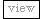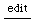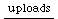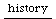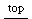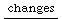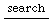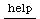## Using VOsc to simulate bandlimited wavetable synthesis

The trouble with wavetable synthesis is that if the wavetable's spectrum is very rich (lots of harmonics), the fundamental frequency must be kept low enough to avoid aliasing. The highest fundamental frequency that will not produce aliasing is the Nyquist frequency divided by the highest partial number. If the wavetable contains 50 partials, an oscillator using that table should not go above 22050 / 50 = 441 or approximately A above middle C. That's a rather small range to be useful.

Alternately, the wavetable could be constructed with a lower number of partials, but that means low-frequency notes would have a dull sound.

What I do instead is build a series of wavetables, each with progressively fewer partials. Then, I map the fundamental on to the appropriate buffer. VOsc interpolates automatically between adjacent buffers so the sound transitions smoothly over several octaves' range.

This function populates the wavetables. Each buffer has half the partials of the preceding buffer, so that one octave maps onto one buffer.

```f = { |numbufs, server, numFrames, lowFreq, spectrumFunc|
numbufs = numbufs ? 8;
server = server ? Server.default;
numFrames = numFrames ? 2048;
// default is sawtooth
spectrumFunc = spectrumFunc ? { |numharm| (1..numharm).reciprocal };
lowFreq = lowFreq ? 131;

Buffer.allocConsecutive(numbufs, server, numFrames, 1, { |buf, i|
var	numharm = (server.sampleRate * 0.5 / lowFreq).asInteger;
lowFreq = lowFreq * 2;
buf.sine1Msg(spectrumFunc.(numharm));
});
};

b = f.value(8, s, 2048);```

Then, the synthdef can use base-two logarithms to determine the right buffer for the frequency. The frequency here sweeps over some five octaves while the perceived spectrum remains a more or less consistent sawtooth.

```a = {
var	freq = LinExp.kr(SinOsc.kr(0.25), -1.0, 1.0, 50, 2000),
basefreq = 131,	// base frequency of first buffer
numOctaves = 7,
numbufs = 8,
// note that subtraction of logs corresponds to division of original values
freqmap = ((log2(freq) - log2(basefreq)) * (numbufs / numOctaves))
.clip(0, numbufs - 1.001),
bufbase = b.first.bufnum;

VOsc.ar(bufbase + freqmap, freq, mul: 0.1) ! 2
}.play;

a.free;```

VOsc3 takes three frequency inputs, allowing easy detuning for fatter sounds.

```a = {
var	freq = LinExp.kr(SinOsc.kr(0.25), -1.0, 1.0, 50, 2000),
basefreq = 131,	// base frequency of first buffer
numOctaves = 7,
numbufs = 8,
// note that subtraction of logs corresponds to division of original values
freqmap = ((log2(freq) - log2(basefreq)) * (numbufs / numOctaves))
.clip(0, numbufs - 1.001),
bufbase = b.first.bufnum;

VOsc3.ar(bufbase + freqmap, freq, freq * 0.997, freq * 1.003, mul: 0.1) ! 2
}.play;

a.free;```

Compare this with Saw.ar, which takes about 50% more CPU (about 1.5% on my macbook pro vs 1% for the VOsc3 example). If you are playing thick chords, the CPU savings of wavetable synthesis becomes really noticeable and leaves you processing power left over for effect processing to get even more richness.

```a = {
var	freq = LinExp.kr(SinOsc.kr(0.25), -1.0, 1.0, 50, 2000);

(Mix(Saw.ar(freq *[1, 0.997, 1.003]))* 0.1) ! 2;
}.play;

a.free;

// clean up buffers when done
b.free;```